Type
Quiz
Book Title
Fundamentals of Corporate Finance Standard Edition 9th Edition
ISBN 13
978-0073382395

### 978-0073382395 Chapter 7 Questions and Problems 25-35

April 3, 2019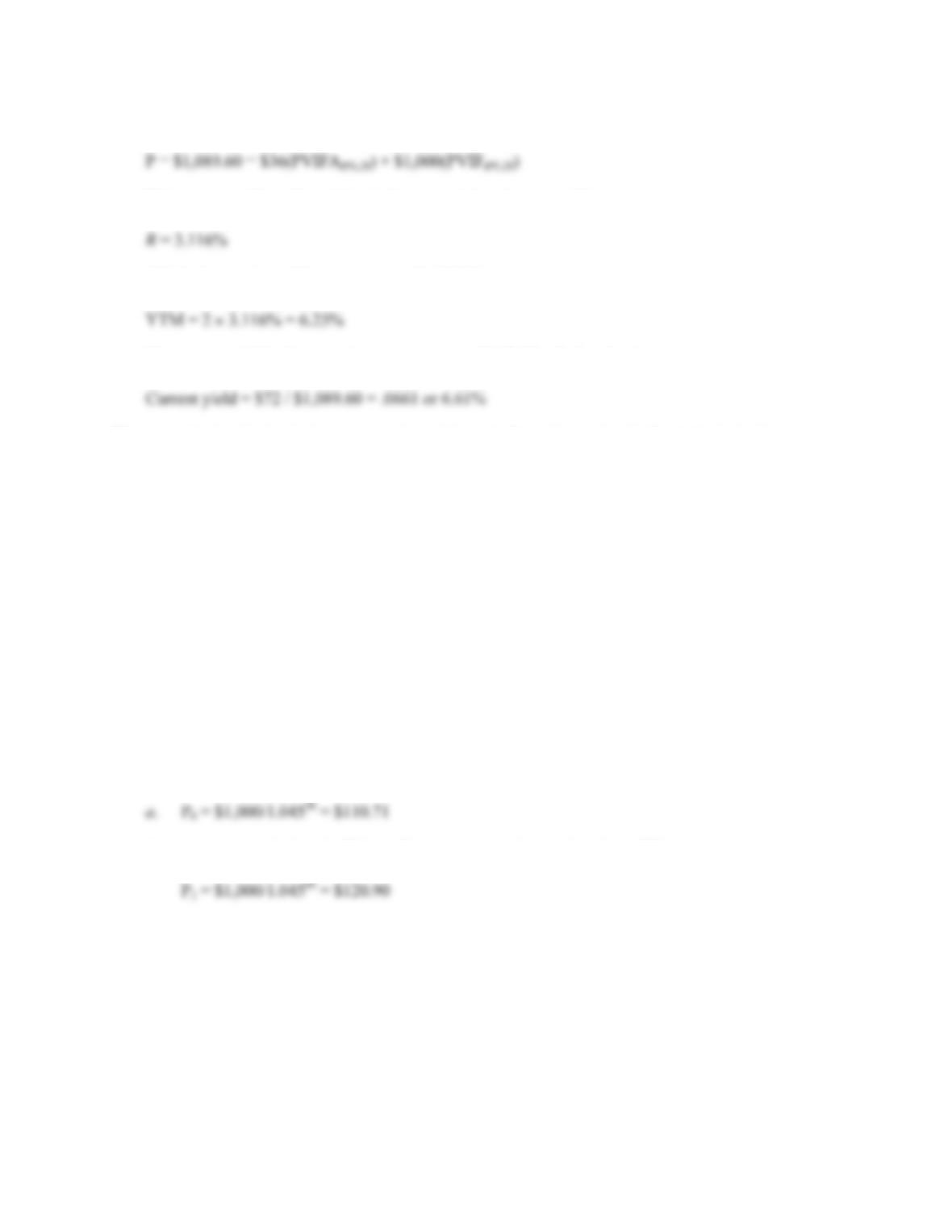CHAPTER 7 B-127
23. The bond has 14 years to maturity, so the bond price equation is:
Using a spreadsheet, financial calculator, or trial and error we find:
This is the semiannual interest rate, so the YTM is:
The current yield is the annual coupon payment divided by the bond price, so:
24. a. The bond price is the present value of the cash flows from a bond. The YTM is the interest rate
used in valuing the cash flows from a bond.
b. If the coupon rate is higher than the required return on a bond, the bond will sell at a premium,
since it provides periodic income in the form of coupon payments in excess of that required by
investors on other similar bonds. If the coupon rate is lower than the required return on a bond,
the bond will sell at a discount since it provides insufficient coupon payments compared to that
required by investors on other similar bonds. For premium bonds, the coupon rate exceeds the
YTM; for discount bonds, the YTM exceeds the coupon rate, and for bonds selling at par, the
YTM is equal to the coupon rate.
c. Current yield is defined as the annual coupon payment divided by the current bond price. For
premium bonds, the current yield exceeds the YTM, for discount bonds the current yield is less
than the YTM, and for bonds selling at par value, the current yield is equal to the YTM. In all
cases, the current yield plus the expected one-period capital gains yield of the bond must be
equal to the required return.
25. The price of a zero coupon bond is the PV of the par, so:
b. In one year, the bond will have 24 years to maturity, so the price will be:
P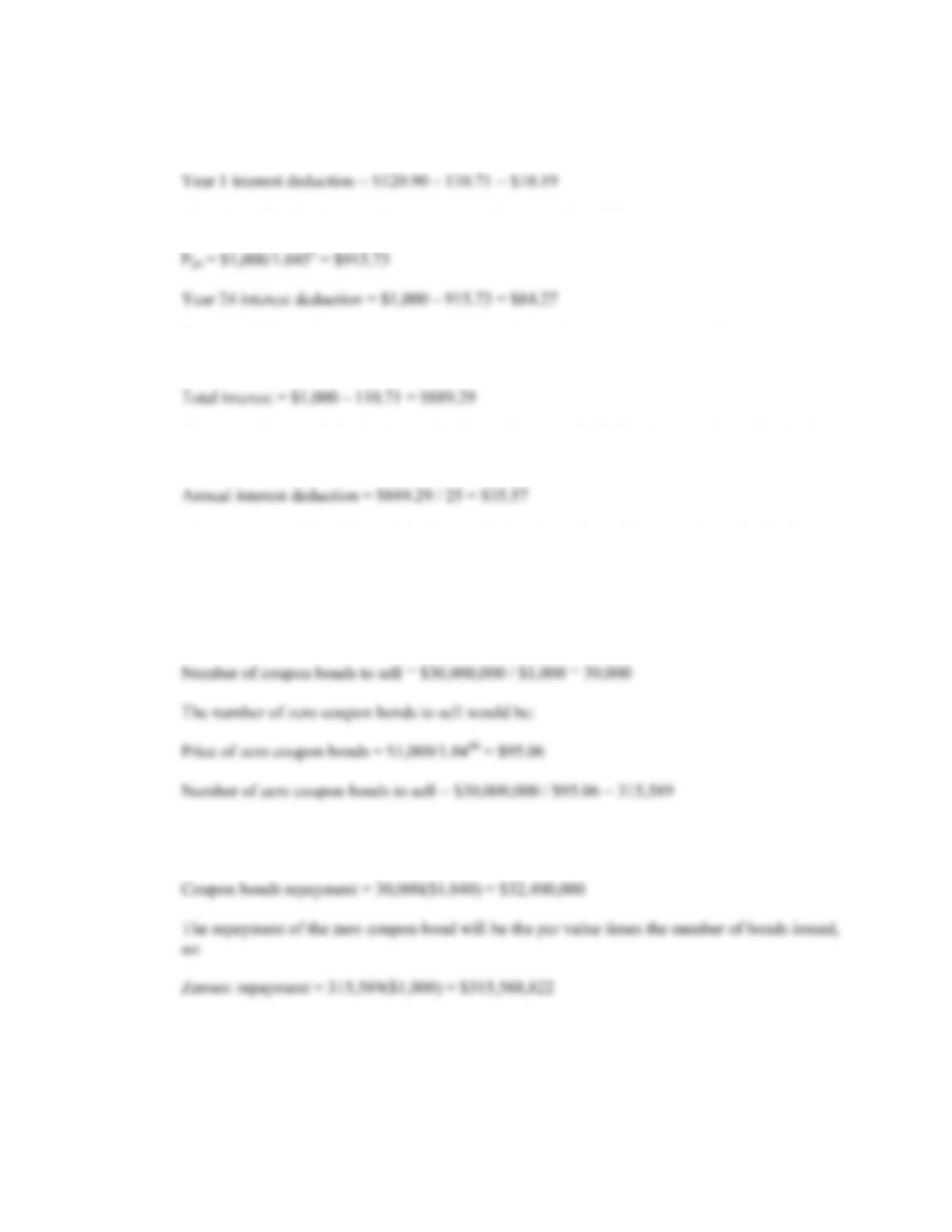B-128 SOLUTIONS
The interest deduction is the price of the bond at the end of the year, minus the price at the
beginning of the year, so:
The price of the bond when it has one year left to maturity will be:
P
c. Previous IRS regulations required a straight-line calculation of interest. The total interest
The annual interest deduction is simply the total interest divided by the maturity of the bond, so
the straight-line deduction is:
d. The company will prefer straight-line methods when allowed because the valuable interest
deductions occur earlier in the life of the bond.
26. a. The coupon bonds have an 8% coupon which matches the 8% required return, so they will sell
at par. The number of bonds that must be sold is the amount needed divided by the bond price,
so:
b. The repayment of the coupon bond will be the par value plus the last coupon payment times the
number of bonds issued. So: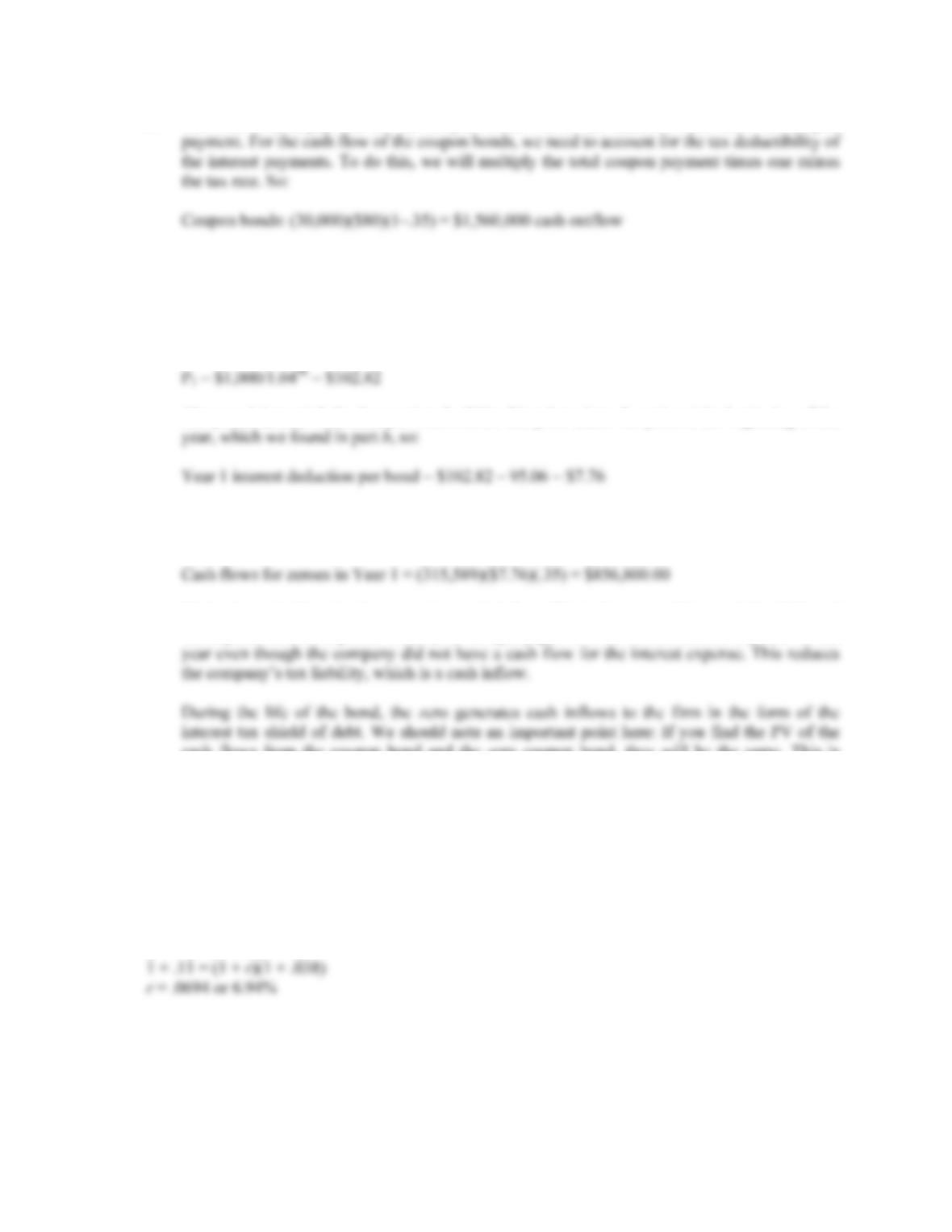CHAPTER 7 B-129
c. The total coupon payment for the coupon bonds will be the number bonds times the coupon
Note that this is cash outflow since the company is making the interest payment.
For the zero coupon bonds, the first year interest payment is the difference in the price of the
zero at the end of the year and the beginning of the year. The price of the zeroes in one year
will be:
P
The year 1 interest deduction per bond will be this price minus the price at the beginning of the
The total cash flow for the zeroes will be the interest deduction for the year times the number of
zeroes sold, times the tax rate. The cash flow for the zeroes in year 1 will be:
Notice the cash flow for the zeroes is a cash inflow. This is because of the tax deductibility of
the imputed interest expense. That is, the company gets to write off the interest expense for the
cash flows from the coupon bond and the zero coupon bond, they will be the same. This is
because of the much larger repayment amount for the zeroes.
27. We found the maturity of a bond in Problem 22. However, in this case, the maturity is indeterminate.
A bond selling at par can have any length of maturity. In other words, when we solve the bond
pricing equation as we did in Problem 22, the number of periods can be any positive number.
28. We first need to find the real interest rate on the savings. Using the Fisher equation, the real interest
rate is:
(1 + R) = (1 + r)(1 + h)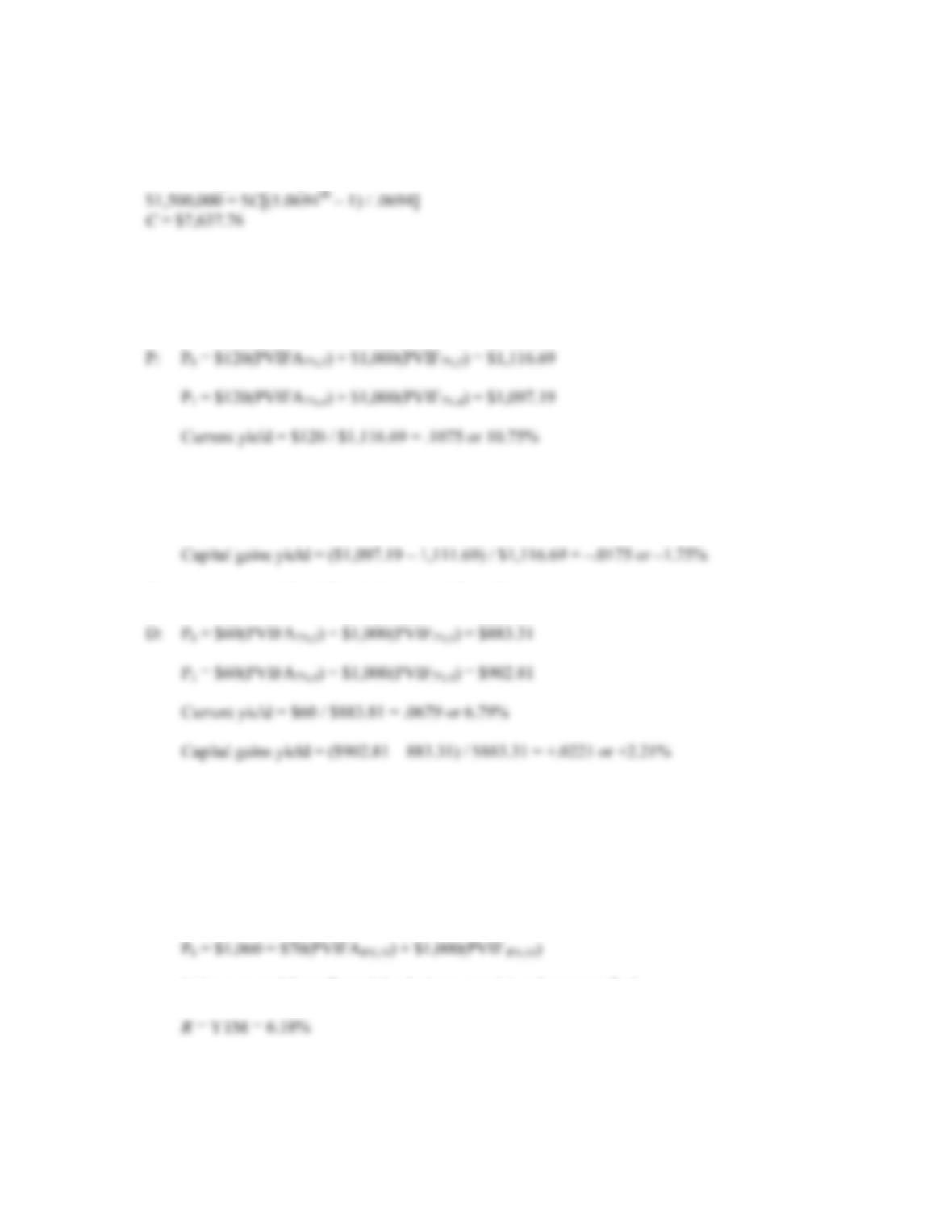B-130 SOLUTIONS
Now we can use the future value of an annuity equation to find the annual deposit. Doing so, we
find:
FVA = C{[(1 + r)t – 1] / r}
Challenge
29. To find the capital gains yield and the current yield, we need to find the price of the bond. The
current price of Bond P and the price of Bond P in one year is:
The capital gains yield is:
Capital gains yield = (New price – Original price) / Original price
The current price of Bond D and the price of Bond D in one year is:
All else held constant, premium bonds pay high current income while having price depreciation as
maturity nears; discount bonds do not pay high current income but have price appreciation as
maturity nears. For either bond, the total return is still 9%, but this return is distributed differently
between current income and capital gains.
30. a. The rate of return you expect to earn if you purchase a bond and hold it until maturity is the
YTM. The bond price equation for this bond is:
P
Using a spreadsheet, financial calculator, or trial and error we find: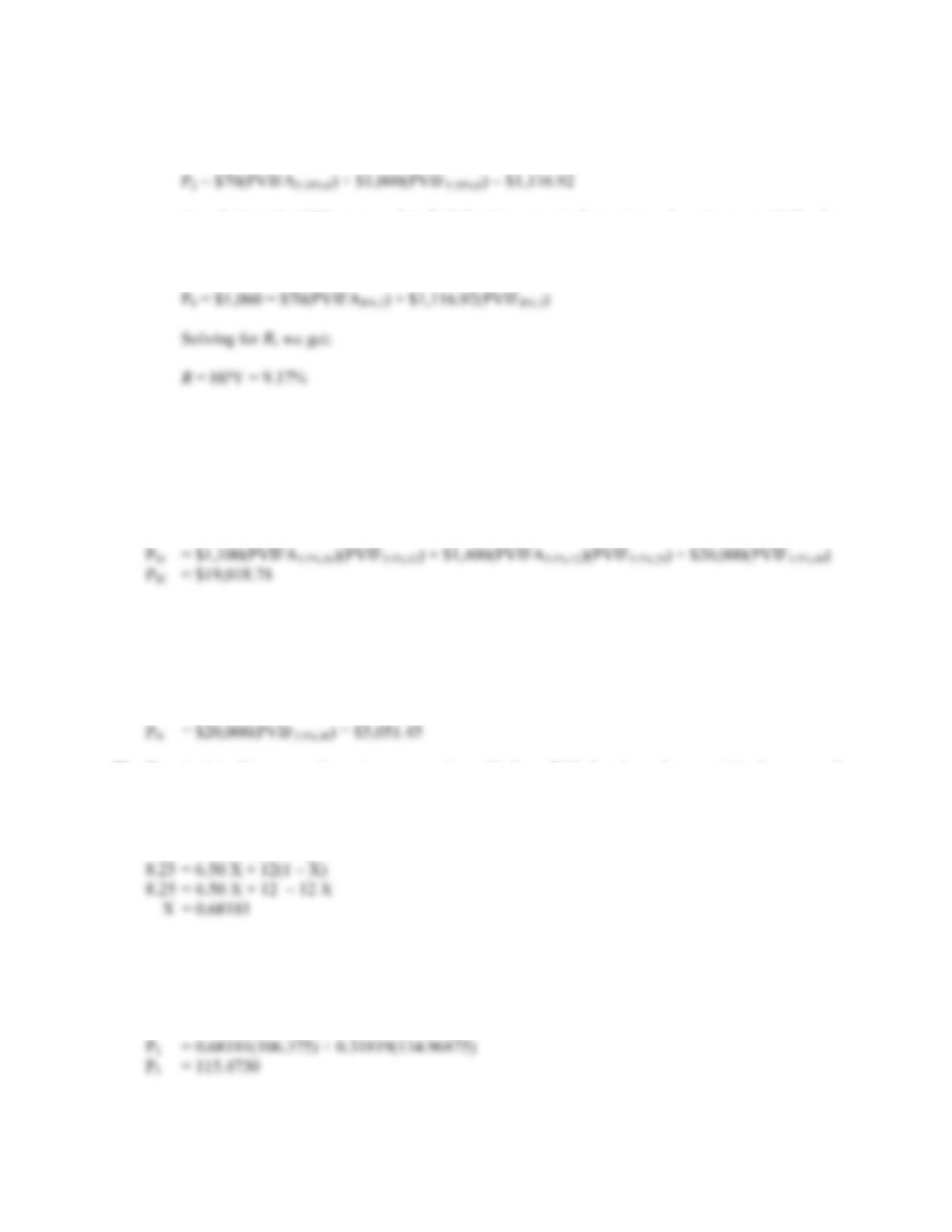CHAPTER 7 B-131
b. To find our HPY, we need to find the price of the bond in two years. The price of the bond in
two years, at the new interest rate, will be:
P
To calculate the HPY, we need to find the interest rate that equates the price we paid for the
bond with the cash flows we received. The cash flows we received were \$70 each year for two
years, and the price of the bond when we sold it. The equation to find our HPY is:
P
The realized HPY is greater than the expected YTM when the bond was bought because interest
rates dropped by 1 percent; bond prices rise when yields fall.
31. The price of any bond (or financial instrument) is the PV of the future cash flows. Even though Bond
M makes different coupons payments, to find the price of the bond, we just find the PV of the cash
flows. The PV of the cash flows for Bond M is:
P
Notice that for the coupon payments of \$1,400, we found the PVA for the coupon payments, and
then discounted the lump sum back to today.
Bond N is a zero coupon bond with a \$20,000 par value, therefore, the price of the bond is the PV of
the par, or:
P
32. To calculate this, we need to set up an equation with the callable bond equal to a weighted average of
the noncallable bonds. We will invest X percent of our money in the first noncallable bond, which
means our investment in Bond 3 (the other noncallable bond) will be (1 – X). The equation is:
C2 = C1 X + C3(1 – X)
So, we invest about 68 percent of our money in Bond 1, and about 32 percent in Bond 3. This
combination of bonds should have the same value as the callable bond, excluding the value of the
call. So:
P
2 = 0.68181P1 + 0.31819P3
P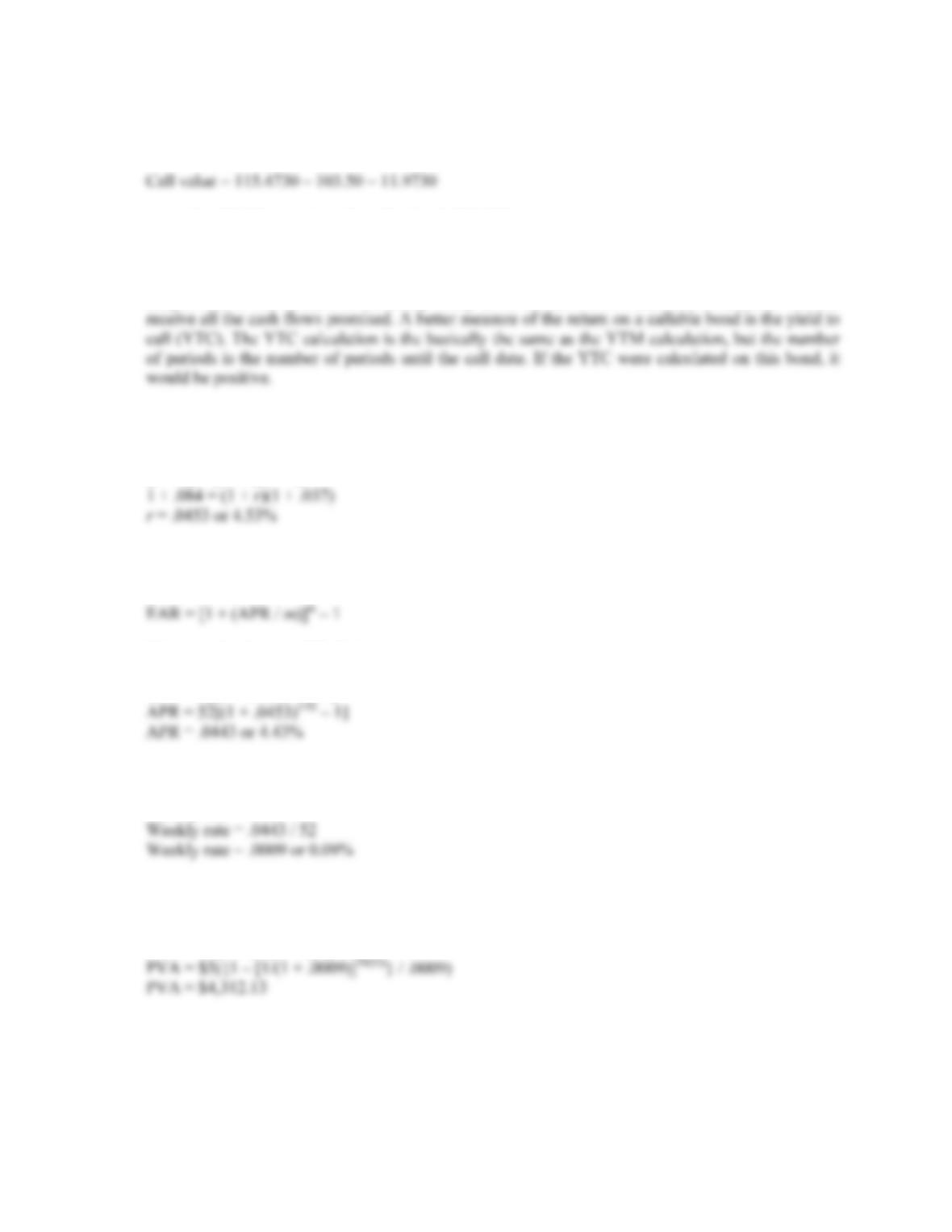B-132 SOLUTIONS
The call value is the difference between this implied bond value and the actual bond price. So, the
call value is:
Assuming \$1,000 par value, the call value is \$119.73.
33. In general, this is not likely to happen, although it can (and did). The reason this bond has a negative
YTM is that it is a callable U.S. Treasury bond. Market participants know this. Given the high
coupon rate of the bond, it is extremely likely to be called, which means the bondholder will not
34. To find the present value, we need to find the real weekly interest rate. To find the real return, we
need to use the effective annual rates in the Fisher equation. So, we find the real EAR is:
(1 + R) = (1 + r)(1 + h)
Now, to find the weekly interest rate, we need to find the APR. Using the equation for discrete
compounding:
We can solve for the APR. Doing so, we get:
APR = m[(1 + EAR)1/m – 1]
So, the weekly interest rate is:
Weekly rate = APR / 52
Now we can find the present value of the cost of the roses. The real cash flows are an ordinary
annuity, discounted at the real interest rate. So, the present value of the cost of the roses is:
PVA = C({1 – [1/(1 + r)]t } / r)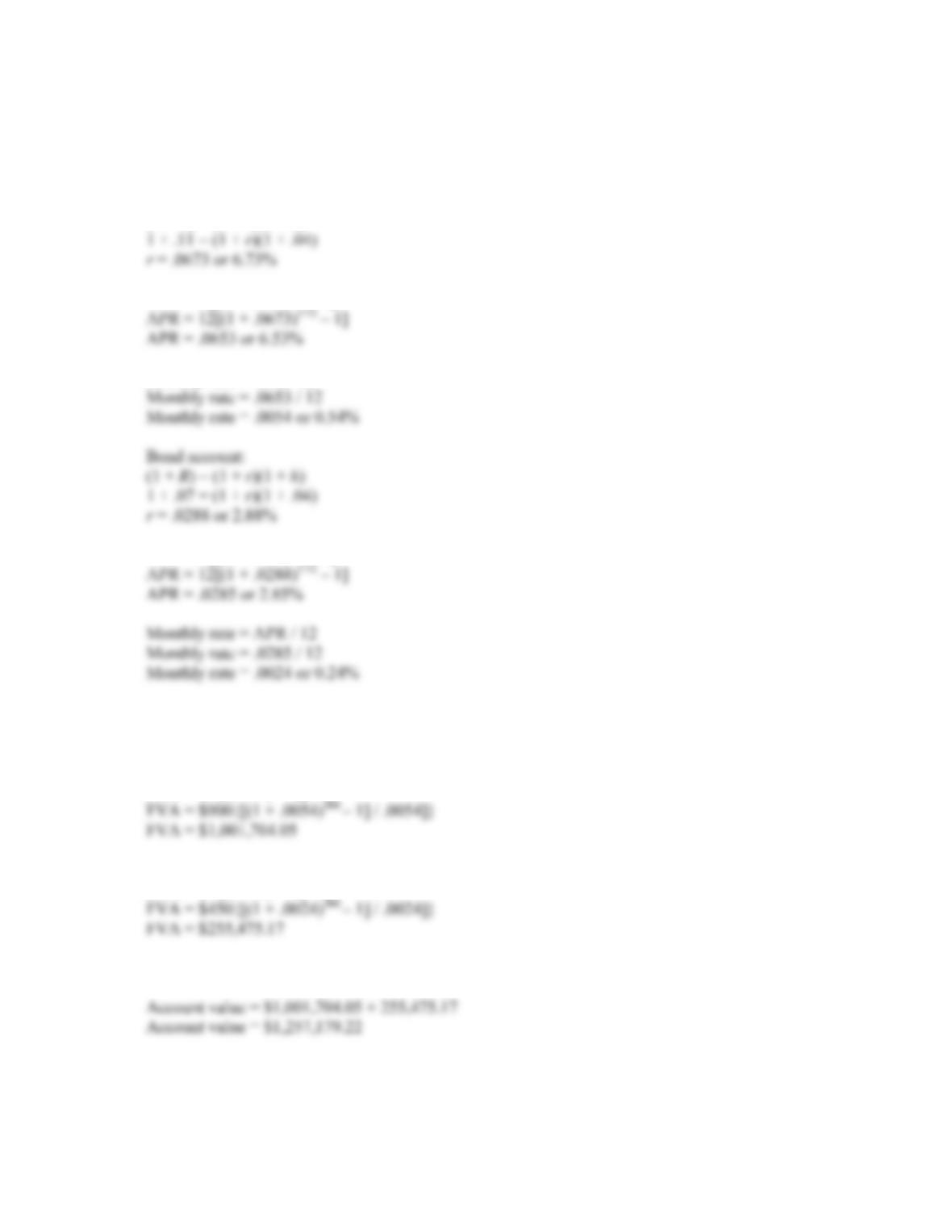CHAPTER 7 B-133
35. To answer this question, we need to find the monthly interest rate, which is the APR divided by 12.
We also must be careful to use the real interest rate. The Fisher equation uses the effective annual
rate, so, the real effective annual interest rates, and the monthly interest rates for each account are:
Stock account:
(1 + R) = (1 + r)(1 + h)
APR = m[(1 + EAR)1/m – 1]
Monthly rate = APR / 12
APR = m[(1 + EAR)1/m – 1]
Now we can find the future value of the retirement account in real terms. The future value of each
account will be:
Stock account:
FVA = C {(1 + r )t – 1] / r}
Bond account:
FVA = C {(1 + r )t – 1] / r}
The total future value of the retirement account will be the sum of the two accounts, or: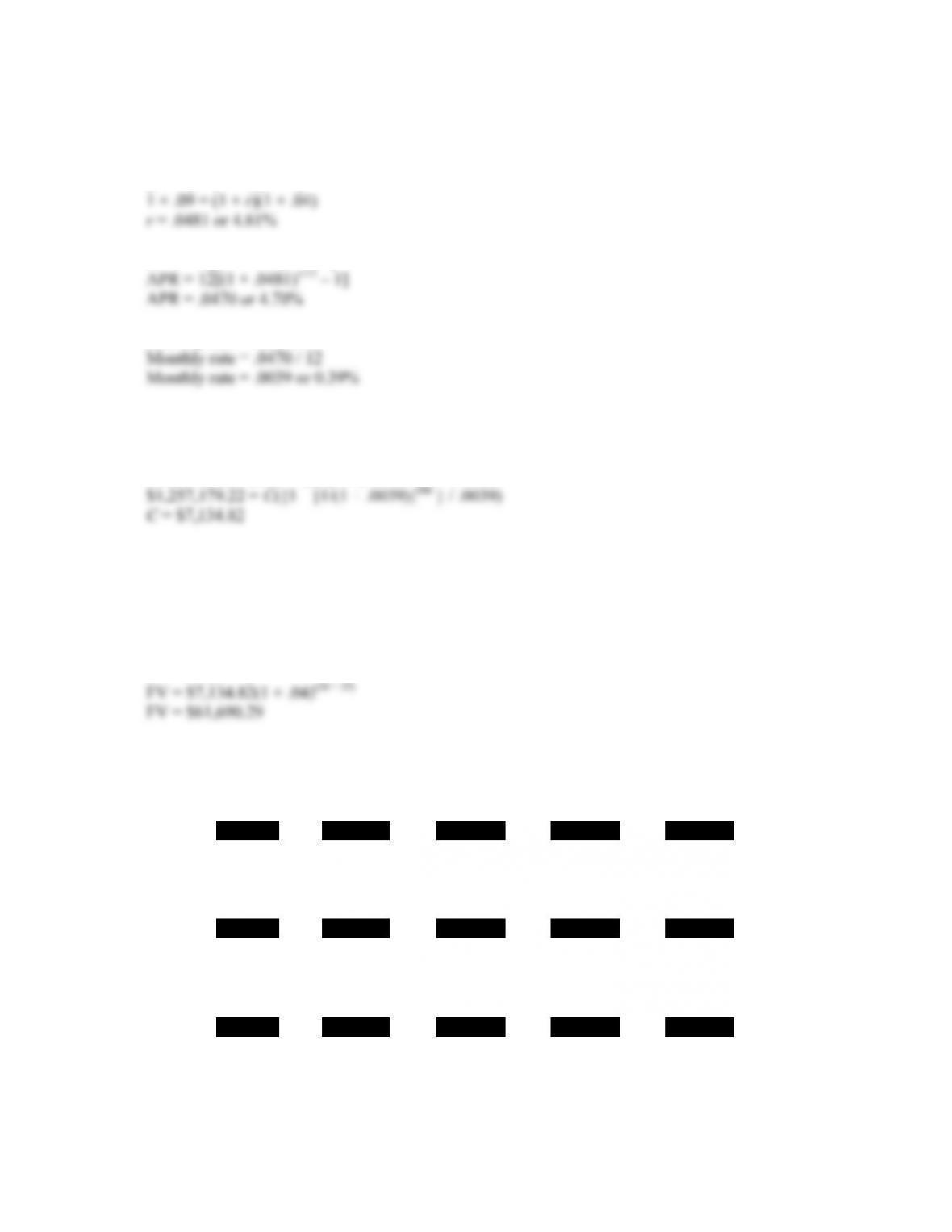B-134 SOLUTIONS
Now we need to find the monthly interest rate in retirement. We can use the same procedure that we
used to find the monthly interest rates for the stock and bond accounts, so:
(1 + R) = (1 + r)(1 + h)
APR = m[(1 + EAR)1/m – 1]
Monthly rate = APR / 12
Now we can find the real monthly withdrawal in retirement. Using the present value of an annuity
equation and solving for the payment, we find:
PVA = C({1 – [1/(1 + r)]t } / r )
This is the real dollar amount of the monthly withdrawals. The nominal monthly withdrawals will
increase by the inflation rate each month. To find the nominal dollar amount of the last withdrawal,
we can increase the real dollar withdrawal by the inflation rate. We can increase the real withdrawal
by the effective annual inflation rate since we are only interested in the nominal amount of the last
withdrawal. So, the last withdrawal in nominal terms will be:
FV = PV(1 + r)t
Calculator Solutions
3.
Enter 10 8.75% \$75 \$1,000
N I/Y PV PMT FV
Solve for \$918.89
4.
Enter 9 ±\$934 \$90 \$1,000
N I/Y PV PMT FV
Solve for 10.15%
5.
Enter 13 7.5% ±\$1,045 \$1,000
N I/Y PV PMT FV
Solve for \$80.54
Coupon rate = \$80.54 / \$1,000 = 8.05%